3. ELECTROCHEMISTRY

Q. 1. How would you determine the standard electrode potential of the system Mg2+/Mg?

Ans⇒ The potential of the system Mg2+ / Mg is determined by constructing a cell in which standard hydrogen electrode is left and electrode and after half cell (Mg2+/Mg) is the right hand electrode. The potential of the other half cell is equal of the potential of the cell. If the activity of all the participating species at the right hand electrode is unity, then the measured potential is called standard potential (E°) of the electrode.

Q. 2. Write the chemistry of recharging the lead storage battery, highlighting all the materials that are involved during recharging.

Ans⇒ Recharging : During charging process, hydrogen ions moves to cathode and sulphate ions to anode and the following reactions take place.

At cathode :
PbSO4 + H2 → Pb + H2SO4
At anode :
PbSO4 + SO4 + 2H2O → PbO2 + 2H2SO4

Thus, during charging active materials namely Pb cut the cathode and PbO2 at the anode are formed. Sulphuric acid is formed and water is consumed. Due to this the specific gravity of sulphuric acid increases and emf of the cell goes up to 2.3 volt.

Q. 3. Explain how rusting of iron is envisaged as setting up of an electrochemical cell.

Ans⇒ At a particular spot of an object made of iron oxidation takes palce and that spot behaves as an anode.

At anode : 2Fe(s) → 2Fe+ 4e
E°(Fe2+, Fe) = 0.44 V

Electrons released at anode spot moves through the metal and go to another spot on the metal and reduce oxygen in presence of H+ (which is believed to be available from H2CO3 formed due to dissolution of carbondioxide from air into water. Hydrogen ion in water may also be available due to dissolution of other acidic oxides from the atmosphere. This spot behaves as a cathode with the reaction.

At cathode :

O2 (g) + 4H+ (aq) + 4e → 2H2O (l) (E° = 1.23 V] The over reaction being

2Fe(s) + O2 (g) + 4H+ (a) → 2Fe2+ (aq) + 2H2O (l) [E° (cell) = 1.67 V]

The ferrous ions are further oxidized by atmospheric oxygen to ferric ions which come out as rust in the form of hydrated ferric oxide (Fe2O3.xH2O)

Q. 4. Depict the galvanic cell in which the reaction

Zn(s) + 2+ Ag (aq) → Zn2+ (aq) + 2Ag (s)

takes place. Further show

(i) Which of the electrode is negatively charged ?
(ii) The carriers of the current in the cell.
(iii) Individual reaction at each electrode.

Ans⇒ The electrochemical cell can be depicted as

Zn (s) | Zn2+ (aq) }} Ag+ (aq)} Ag (s)
Anode                            Cathode
(- ve)                                 (+ ve)

(i) Cathode reaction :

2Ag+ (aq) + 2e → 2Ag(s)

(positive electrode reduction)

Anode reaction :

Zn(s) → Zn2+ (aq) + 2e

(negative electrode oxidation)

(ii) Electrons move from anode (zinc electrode) to cathode (silver electrode) in the external circuit. zinc ions go into solution at anode and Ag+ ions get deposited cathode. Thus electrons in the external and metal ions in the internal circuit act as carrier of current in

Zn(s) + 2Ag+ (aq) → Zn2+ (aq) + 2Ag (s)

(iii) Overall reaction is obtained by anode and cathode reactions.

Q. 5. What is the Nernst equation for the Potential of an electrode ? Can Nemst equation be applied to the cell relation ? Apply this equation to a general reaction.

aA + bB → cC + dD

Ans⇒ Let us take a electrode reaction

Zn2+ + 2e → Zn

The Nernst equation of this electrode

E = E° –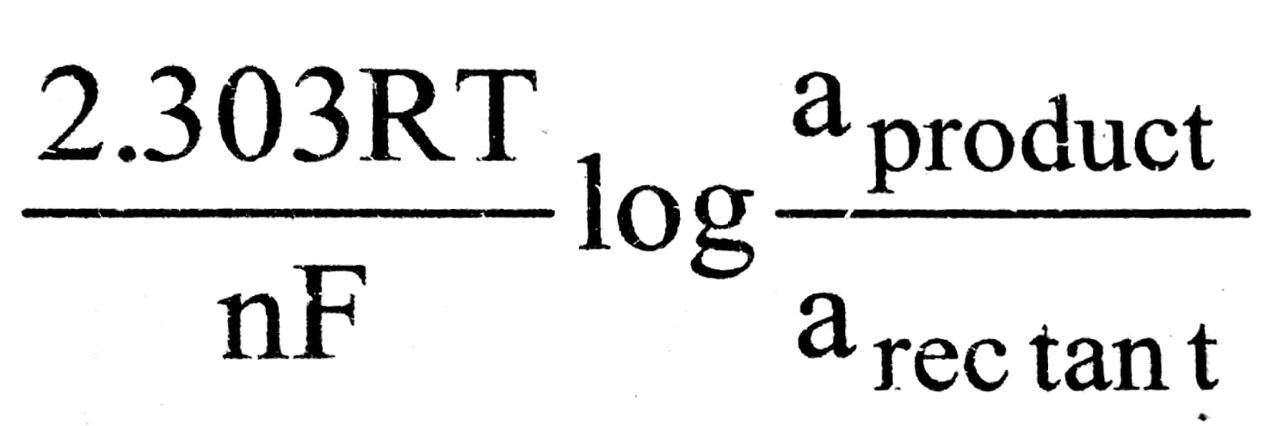Instead of activity, we can take molar concentration.

E = E° –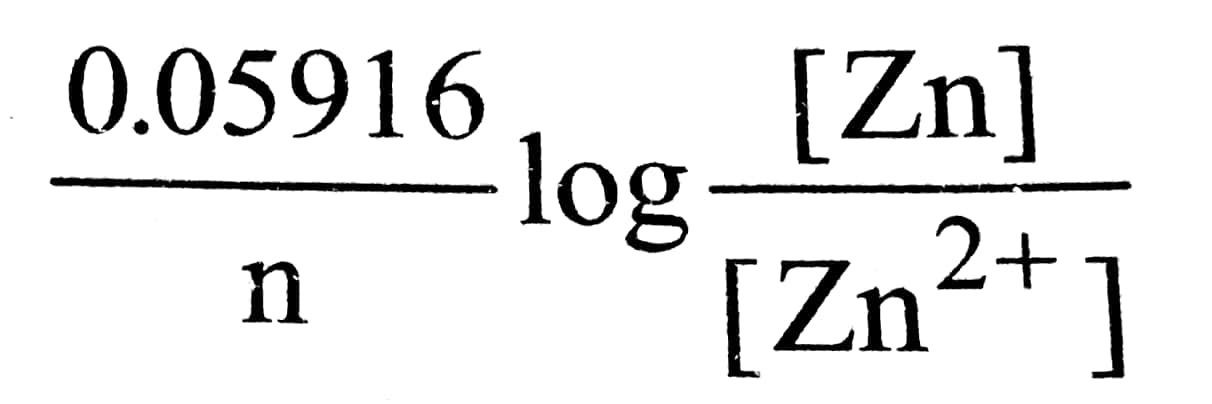For pure solid and liquid molar concentration is taken as unity.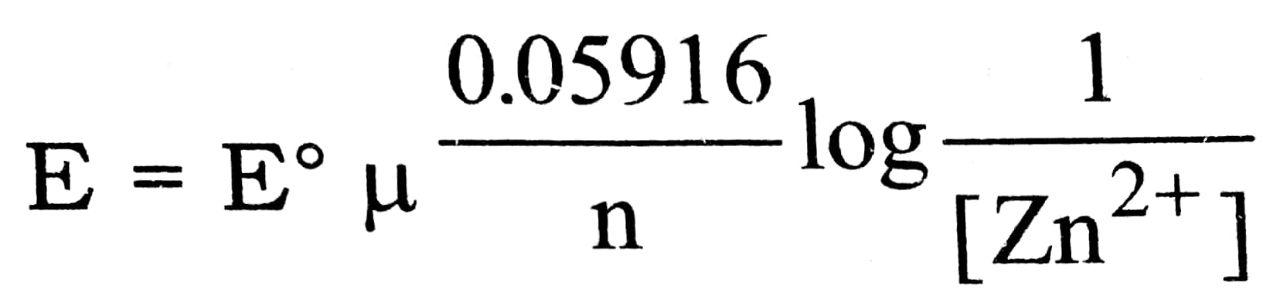Yes, Nernst equation can be applied to the cell reaction.

aA + bB → cC + dD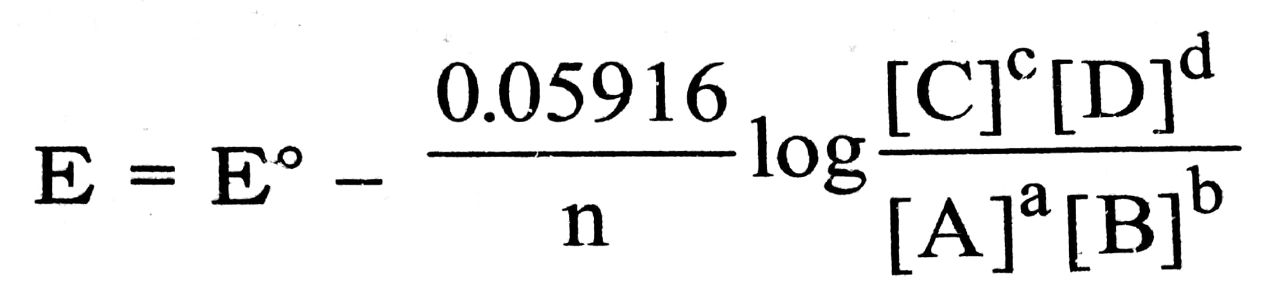Q. 6. State and explain Faraday’s law of electrolysis.

Ans⇒ Faraday’s First law : The amount of substance produced at an electrode during electrolysis is directly proportional to the quantity of electricity passed through the electrolyte.

.            W  ∞  Q
or,        W  ∞  It
or,        W  ∞  Zlt

where W is the mass of substance produced at an electrode.

I is current in amperes
t is time in seconds for which current is passed.
Z is electro-chemical equivalent of substance.

Faraday’s second law : It states that the masses of different substances liberated or dissolved by the same amount of electricity passed is directly proportional to their chemical equivalents. Or in other words “the same quantity of electricity will produce or dissolve chemically equivalent quantities of all substances.”

Mathematically,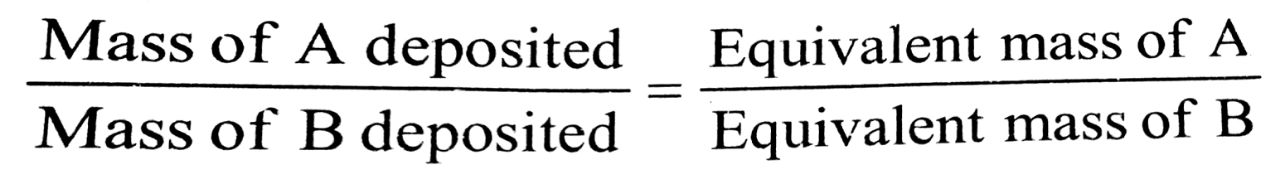Thus, we can say that the same quantity of Blectricity is required to produce one equivalent of any
hstance. It is called Faraday, F. It is equal to 96500 coulombs, and is equal to the charge on one mole of electrons.

Q. 27. What are fuel cells ? Write the reaction of a oxygen hydrogen fuel cell.

Ans Fuel Cell : A galvanic cell in which the reactants are continuously fed into the cell and the products are continuously removed is called a fuel cell

The most important fuel cell is hydrogen oxygen fuel cell.

The reactions taking place in this fuel cell are
At cathode :
O2 + 2H2O (l) + 4e → 40H E0cathode = 1.299 V
At anode :
2H2 (g) + 40H → 4H2O + 4e E0cathode = 0.00 V
Net reaction :
02 + 2H2 → 2H20 E0cell = 1.299 V

Q. 8. What is mercury cell ? Give the electrode reactions.

Ans⇒ Mercury cell is new type of dry cell. It consists of

Anode : Zinc-mercury
Cathode : Paste of HgO and carbon
Electrolyte : Paste of ZnO in KOH. The reactions are :
Anode : Zn (amalgam) + 2OH → ZnO(s) + H2O + 2e
At cathode : HgO + H2O + 2e → Hg (l) + 2OH
Overall reaction : Zn (amalgam) + HgO (s) → ZnO (s) + Hg (l)

Q. 9. Define the terms equivalent and molar conductivity. What are their physical significance ?

Ans⇒ Equivalent conductivity : The conductivity of a volume (V) of a solution containing one equivalent of electrolyte placed between two electrodes separed by unit distance apart and of large enough area of cross section to hold the entire volume (V) is called equivalent conductance. It is denoted by ^eq.

^eq = K × V

where K = Specific conductance
V = Volume of solution containing one equivalent of electrolyte.

Molar conductivity : It is the conductivity of volume (V) of a solution containing 1 mole of a dissolved electrolyte place between two electrodes separated by unit distance apart and of enough area of cross-section to hold the entire volume V. It is denoted by Am .

^m = K × V = K/V
where V = Volume of solution
Containing 1 mole of electrolyte
C = molarity of solution
K = Specific conductivity.

Q. 10. Define entropy. Why is it a state function ? Explain the effect of in increased temperatures on the entropy a substance.

Ans⇒ Entropy is a measure of the degree of randomness or disorder of a system. It is denoted by S. It is state function and a change in state of a system is accompanied by a change in entropy when disorder increases entropy also increases.

Entropy change in reversible process.

According to Carnot’s cycle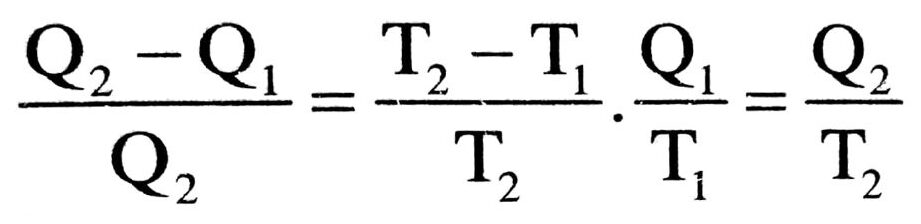or,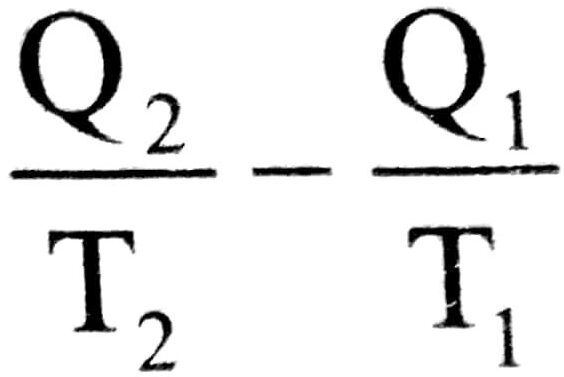= 0 on quantity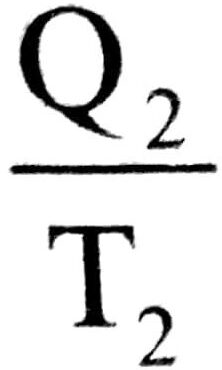and T2 are change in entropy and it is denoted by ΔS2.
Similarly onand T1 are less in entropy and it is denoted by ΔS1 .
∴ ΔS2 – ΔS1 = o
Hence in reversible reaction or process entropy of the system is constant ΔS = 0∫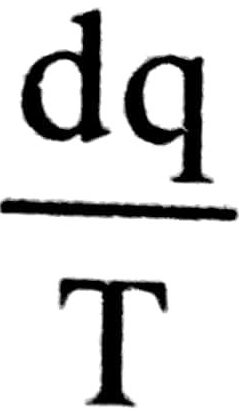= 0 .

Q. 11. (i) What is corrosion ? What are the factors which affect corrosion ?
(ii) CO2 is always present in natural water. Explain its effect (increases, stops or no effect) on rusting of iron.
(iii) Rusting of iron is quicker in saline water than in ordinary water. Explain.
(iv) We can use aluminium in place of zinc for cathodic protection of rusting, Comment.
(v) How is cathodic protection of iron different from its galvanisation?

Ans⇒ (i) The gradual eating away of metals exposed to the atmosphere is known as corrosion. The phenomenon of corrosion involves the destruction of metal in which metal is generally converted into oxide. Common examples are rusting of iron, tarnishing of silver and deposition of green coating on copper and bronze.

Factors affecting corrosion :

(a) Presence of oxygen, sulphur etc. (elements which gain electrons).
(b) presence of moisture.
(c) Presence of carbon dioxide.
(ii) CO2 increases rusting of iron : CO2 present in water is in the form of H2CO3, H2CO3 dissociates into H+ and HCO3.

H2CO3 = HCO3 = H+

H2O = H+ + OH
2H+ + 2e →H2

Hydroxyl ion or bicarbonate ions attack the iron surface to form aniodic regions in which iron loses electrons and pass on to ferrous sulphate which is further oxidised to ferric state by oxygen of the air. The released electrons moved towards the cathode region when H+ ions are converted into hydrogen gas.

Fe → Fe2+ + 2e
O2 = 2H2O + 4e → 4OH
4Fe + 3O2 + 2 × H2O → 2Fe2O3. XH2O

Thus CO2 increases rusting because H2CO3 gives H+ which gain electrons to form H2 gas. The electrons are released by iron.

(iii) Saline water contains greater number of irons Taissolved salts, NaCl) than ordinary water and therefore ectro-chemical reaction is quicker with saline water man when ordinary water is present. This increases rate of corrosion of metal.

(iv) We can use aluminium in place of zinc for cathodic protection because aluminium is more reactive than Fe. When a plate of Al metal is buried inside the iron pipe nor tank and connected to it by wires. The Al metal loses electrons easily and is easily sacrificed and protects from rusting

At anode : Al → Al3+ 3e
At cathode : O2 + 2H2O + 4e 4OH

(v) In cathode protection, the iron object is made cathode by connecting it with a more reactive metal like Mg, Zn etc. Where as in galvanisation, the iron object is protected from corrosion by coating it with zinc.

Q. 12. Explain Kohrausch’s law of independent migration of ions. Mention one application of this law ?

Ans⇒ At infinite dilution when the dissociation is complete each ion of the electrode make a definite contribution of its own towards the molar conductivity of the electrolyte and it is quite independent of the presence of the other ion of the electrolyte. This the molar conductivity of an electron lyte at infinite dilution is sum of the ionic conductive of the cations and anions i.e.

λ0m = λ + λ

Q. 13. Why does conductivity of a solution decrease with dilution ?

Ans⇒ The conductivity of a solution is linked with the number of ions per unit volume with dilution these decrease and the corresponding conductivity or specific conductance of the solution decreases.

Q. 14. Define Kohlrausch’s law. How does it help in

(i) calculating of Δ for a weak electrolyte.
(ii) degree of dissociation of a weak electroyte.

Ans⇒ “The rnolar conductivity of an electrolyte at infinite dilution is the sum of the ionic conductivities of the cations and anions each multipled with the number of ions present in one formula unit of the electrolyte.”

Mathematicallyfor AxBy = xλy+ + yλx

whereis the molar conductivity of the electrolyte

at infinite dilution λy and λx are the molar conductivities of the cation Ay+ and the anion Bx respectively at infinite dilution.

For examplefor NaCl = λNa ++ λClfor BaCl2 = λBa2++ λCl-for Al2(SO4)3 = 2λ∝Al3+ 3λ∝SO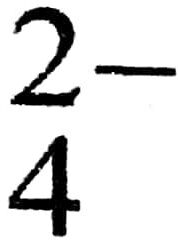In term of equivalent conductivities, Kohlrausch’s law is defined as follows. “The equivalent conductivity of an electrolyte at infinite dilution is the sum of two values-one depending upon the cation and the other upon anions.”

i.e.,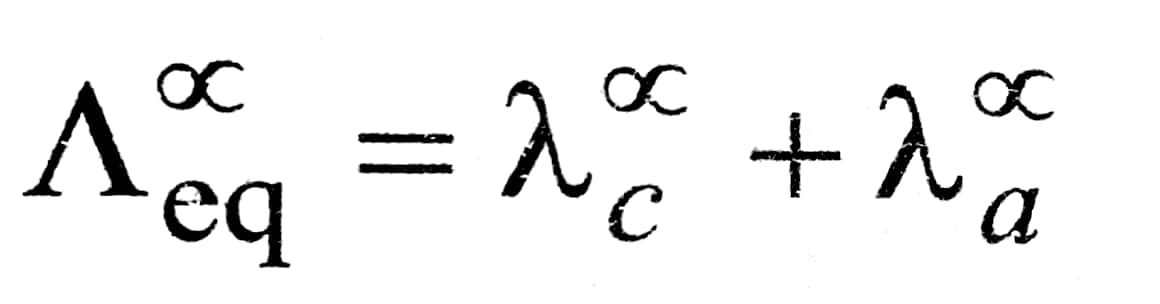where λandare called the ionic conductivities at infinite dilution for the cation and anion respectively.

Applications of Kohlrausch’s Law : Some of the important applications of Kohlrausch’s law are :

1. Calculation of molar conductivities of weak electrolytes at infinite dilution : Kohlrausch’s law is helpful in determining the limiting molar conductivities of weak electrolytes. It has been discussed earlier the value of ∧for weak electrolytes cannot be obtained directly by the extrapolation of plot of ∧m versus √c . But this can be easily calculated with the help of Kohlrausch’s law.

For example, the value of ∧for acetic acid (CH3COOH) can be calculated from the knowledge of the molar conductivities at infinite dilution of strong electrolytes like CH3COONa, HCl and NaCl as follows :(CH3COOH) = λ (CH3cCOO-) + λ (H+)

Now, add and subtract λ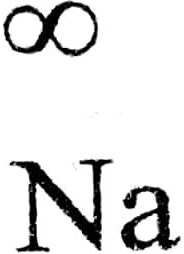+ and λ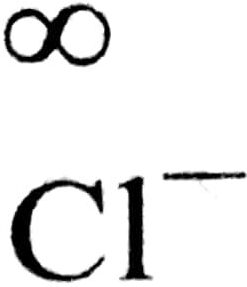to the expression on right hand side and rearrange.

Thus, ∧∞(CH3COOH) = λ CH3COO- + λH++ -λ+=[λ CH3COO+ λ+] +[λ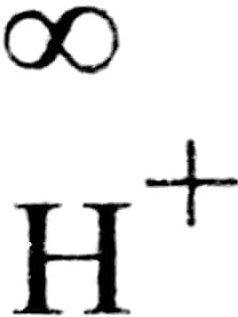λ] – [ λ+]

=(CH3COONa)+(HCl)(NaCl)

In the similar way ,(HN4OH) =(NH4Cl) +(NaOH)(NaCl)

2. Calculation of degree of dissociation of weak electrolyte : Molar conductivity of an electrolyte depends upon its degree of dissociation. Higher the degree of dissociation, higher is the molar conductivity. As dilution increases, the degree of dissociation of weak electrolyte also increases and consequently, molar conductivity of electrolyte increases. At infinite dilution molar conductivity becomes maximum because degree of dissociation approaches unity.

Thus, if ∧cm= molar conductivity of solution at any concentration.= molar conductivity at infinite dilution
α = degree of dissociation

α =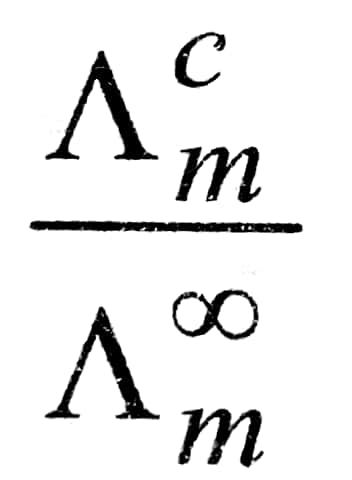Q. 15. (a) Distinguish between Electrolytic cell and Electrochemical cell.
(b) E.M.F. and Potential difference.
(c) Metallic conduction and Electrolytic conduce conduction.

Ans⇒ distinction between Electrolytic and Electro chemical cell or (Galvanic cell) :

 Galvanic cell Electrolyte cell 1. In galvanic cell,electrical energy is produced. 1. In electrolytic cell, electrical energy is Consumed. 2. In galvanic cell,reaction taking place is spontaneous. 2. In electrolytic cell, reaction taking place is non-spontaneous. 3. The two half cell are set up in different containers and are connected through salt bridge or porous partition. 3. Both the electrodes are placed in the solution or| molten electrolyte in the same container. 4. In galvanic cell, anode 1s negative and cathode is positive. 4. In electrolytic cell, the anode is positive and cathode is negative. 5. The electrons move from anode to cathode in external circuit. 5. The electrons are supplied by the external Source. They enter through cathode and come out through anode.

(b) Distinction between Potential defference and E.M.F. of the cell:

 Potential difference E.M.F. 1. It is the difference of potential between the two electrodes when the cell is producing current iie., the circuit is close. 1. It is the difference of potential between the two electrodes when no net current flows through the cell i.e. when the circut is open. 2. It is always less than the e.mn.f. of the cell. 2. It is the maximum potential difference between the two electrodes of the cell. 3 It does not have a definite value and varies as the cell produces electricity. The work calculated 3. It has a definite value of the cell 4. from the potential difference is always less than the maximnum work obtainable from the cell. 4. The maximum that can be obtained from a cell is calculated from its e.m.f.

(c) Distinction between Metallic conduction and Electrolytic conduction

 Metallic Conduction Electrolytic Conduction 1. Conduction through metals is carried by the movement of electrons. 1 Conduction through electrolytes is carried by movement of ions. 2. No change is observed 2. in the chemical properties of the conductors. 2. It involves chemical change resulting in the decomposition of electrolytes. 3. It does not involve any transfer of matter. 3. It involves the transfer of matter as ions. 4. The resistance of metals is due to obstacles of vibrating kernels of metal atoms. 4. The resistance of solution of electrolyte is due to factors like inter-inoic attraction, viscosity of solvent etc. 5. It shows increase in resistance with the increase in temperature. 5. It shows decrease in resistance with the increase in temperature. 6. The conductivity of metals is generally high. 6. The conductivity of electrolytic solutions is generally low.

## Class 12th Chemistry Long Type Question

 S.N CHEMISTRY LONG TYPE QUESTION 2022 1 SOLID STATE 2 SOLUTION 3 ELECTROCHEMISTRY 4 CHEMICAL KINETICS 5 SURFACE CHEMISTRY 6 GENERAL PRINCIPLES AND PROCESSES OF ISOLATION OF ELEMENTS 7 THE P-BLOCK ELEMENTS 8 THE D- AND f-BLOCK ELEMENTS 9 CO-ORDINATION COMPOUNDS 10 HALOALKANES AND HALOARENES 11 ALCOHOLS, PHENOLS AND ETHERS 12 ALDEHYDES, KETONES AND CARBOXYLIC ACIDS 13 AMINES 14 BIOMOLECULES 15 POLYMERS 16 CHEMISTRY IN EVERYDAY LIFE 17 DISTINGUISH BETWEEN PAIR 18 CONVERSIONS